# Samacheer Kalvi 7th Science Solutions Term 1 Chapter 3 Matter Around Us

Students can Download Science Chapter 3 Matter Around Us Questions and Answers, Notes Pdf, Samacheer Kalvi 7th Science Book Solutions Guide Pdf helps you to revise the complete Tamilnadu State Board New Syllabus and score more marks in your examinations.

## Tamilnadu Samacheer Kalvi 7th Science Solutions Term 1 Chapter 3 Matter Around Us Question and Answer

### Samacheer Kalvi 7th Science Matter Around Us Textual Evaluation

I. Choose the appropriate answer :

Matter Around Us Class 7 Question 1.
Which of the following is an example of a metal?
(a) Iron
(b) Oxygen
(c) Helium
(d) Water
(a) Iron

7th Standard Science Matter Around Us Question 2.
Oxygen, hydrogen, and sulphur are examples of which of the following?
(a) Metals
(b) Non-metals
(c) Metalloids
(d) Inert gases
(b) Non-metals

7th Science Matter Around Us Question 3.
Which of the following is a short and scientific way of representing one molecule of an element or compound?
(a) Mathematical formula
(b) Chemical formula
(c) Mathematical symbol
(d) Chemical symbol
(d) Chemical symbol

7th Standard Science Matter Around Us Question Answer Question 4.
The metals which is a liquid at room temperature
(a) Chlorine
(b) Sulphur
(c) Mercury
(d) Silver
(c) Mercury

Matter Around Us 7th Standard Question 5.
An element which is always lustrous, malleable and ductile
(a) non-metal
(b) metal
(c) Metalloid
(d) gas
(b) metal

II. Fill in the blanks :

1. The smallest particle of matter that can exist by itself ______
2. A compound containing one atom of carbon and two atoms of oxygen is ______
3. ______ is the only non-metal conducts electricity.
4. Elements are made up of ______ kinds of atoms.
5. ______of some elements are derived from Latin or Greek names of the elements.
6. There are ______ number of known elements.
7. Elements are the ______ form of pure substances.
8. The first letter of an element always written in ______ letter.
9. Molecule containing more than three atoms are known as ______
10. ______ is the most abundant gas in the atmosphere.

1. atom
2. CO2
3. Graphite
4. same
5. Symbol
6. 118
7. simplest
8. capital
9. polyatomic molecule
10. Nitrogen

III. Fill in the blanks :

1. Mercury: liquid at room temperature:: Oxygen: _________
2. Non metal conducting electricity: _______ :: Metal conducting electricity: Copper
3. Elements: combine to form compounds:: Compounds: ________
4. Atoms: fundamental particle of an element:: ________ fundamental particles of a compound

1. Gas at room temperature
2. Graphite
3. can be split into elements
4. elements

IV. True of False. If False, give the correct statement.

7th Standard Matter Around Us Question 1.
Two different elements may have similar atoms.
True

Matter Around Us Class 7 Question Answer Question 2.
Compounds and elements are pure substance.
True

Samacheer Guru 7th Science Question 3.
Atoms cannot exist alone; they can only exist as groups called molecules.
True

Samacheer Kalvi Guru 7th Science Question 4.
NaCl represents one molecule of sodium chloride.
True

Matter Around Us Questions And Answers Question 5.
Argon is mono atomic gas.
True

Samacheer Kalvi Guru Science 7th Question 1.
Write the chemical formula and name the elements present in the following compounds:
a. Sodium chloride
b. Potassium hydroxide
c. Carbon-di-oxide
d. Calcium oxide
e. Sulphur dioxide

 Chemical fromula Elements present a. Sodium chloride NaCl Sodium (Na), Chlorine (Cl) b. Potassium hydroxide KOH Potassium (K), Oxygen (O), Hydrogen (H) c. Carbon-di-oxide CO2 Carbon (C), Oxygen (O2) d. Calcium oxide CaO Calcium (C), Oxygen (O2) e. Sulphur dioxide SO2 Sulphur (S), Oxygen (O2)

Kalvi Guru 7th Standard Question 2.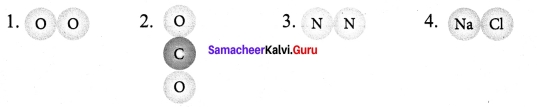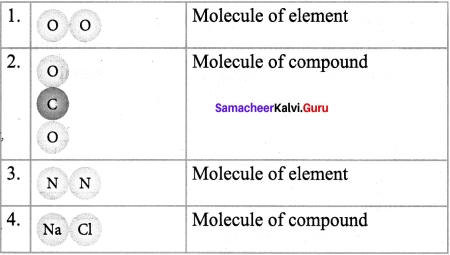Samacheer Kalvi Guru 7th Science Book Back Answers Question 3.
What do you understand by chemical formula of a compound? What is its significance?

1. A chemical formula is a symbolic representation of one molecule of an element or a compound.
2. It provides information about the elements present in the molecule and the number of atoms of each element. The chemical formula tells us the types of atoms and the number of each type of atom in one molecule of substance.

Samacheer Kalvi.Guru 7th Science Question 4.
Define the following terms with an example of each:
a. Element
b. Compound
c. Metal
d. Non-metal
e. Metalloid
(a) Element: It is a substance that cannot be broken down into simpler substance by chemical means Ex. : Oxygen, Hydrogen, Gold & Helium.

(b) Compound A compound is a pure substance that is formed when the atoms of two or more elements combine chemically in definite proportions. Ex : H?0, NaCl.

(c) Metal A chemical element that is an effective conductor of electricity and heat can be defined as a metal. Ex.: Copper, Iron, Silver, etc.
(d) Non-Metal Non-metal is an element that doesn’t have the characteristics of metal including, (i.e.) ability to conduct heat or electricity luster or flexibility. Ex. Carbon Iodine, Sulphur.

(e) Metalloid : Metalloid is a chemical element that exhibits some properties of metals and some of non-metals. Metalloids are generally semi-conductors. Ex. : Silicon. Arsenic, Antimony and Boron.

Samacheerkalvi.Guru 7th Science Question 5.
Write the symbols for the following elements and classify them as solid, liquid and gas.
Aluminum, carbon, chlorine, mercury, hydrogen and helium.

 Element Symbol Classification Aluminum Al Solid Carbon C Solid Chlorine. Cl Gas Mercury. Hg Liquid Hydrogen. H Gas Helium He Gas

Samacheer Kalvi Guru 7th Standard Science Question 6.
Classify the following as metals, non-metals and metalloids
Sodium, Bismuth, Silver, Nitrogen, Silicon, carbon, chlorine, Iron, copper.

 Metal Non-metal Metal Non-metal Sodium Metal Carbon Non-metal Bismuth Metal Chlorine Non-metal Silver Metal Iron Metal Nitrogen Non-metal Copper Metal Silicon Metalloid

Samacheer Kalvi Guru 7th Science Guide Question 7.
Classify the following as elements and compounds.
Water, common salt, sugar, carbon dioxide, iodine and lithium

 Elements Compounds Water Compound Common salt compound Sugar Compound Carbon dioxide Compound Iodine Element Lithium Element

7th Science Solutions Samacheer Kalvi Question 8.
Write the chemical formula for the following elements.
a. Hydrogen
b. Nitrogen
c. Ozone
d. Sulphur

 Elements Formula Hydrogen H Nitrogen N Ozone O3 Sulphur S

Matter Around Us Meaning In Tamil Question 9.
What are elements? What are they made of. Give two examples.

1. Elements are chemically the simplest substances and hence cannot be broken down using chemical reactions.
2. It is made of entirely from one type of atom.
3. Example : Hydrogen, Oxygen is made from atoms containing a single proton and a single electron.

Samacheer Kalvi 7th Science Solutions Question 10.
Define molecule.

1. When an atom combines with another atom (or atoms) and forms a compound it is called as molecule.
2. A molecule is made up of two or more atoms chemically combined.

Samacheer Kalvi 7th Science Solutions Term 1 Question 11.
What are compounds? Give two examples.
A compound is a pure substance that is formed when the atoms of two or more elements combine chemically in definite proportions.
Example : H2O, NaCl,

Matter Around Us Book Back Answers Question 12.
Give an example for the elements derived from their Latin names.

 Element Latin Name Symbol Copper Cuprum Cu Lead Plumbum Pb Potassium Kalium K Iron Ferrum Fe Mercury Hydrargyrum Hg Sodium Natrium Na

Samacheer Kalvi 7th Standard Science Solution Question 13.
What is atomicity of elements?
Atomicity is the total number of atoms present in one molecule of an element, compound or a substance.

Matter Around Us Class 8 Samacheer Question 14.
Calculate the atomicity of H2S04.
A molecule of sulphuric acid (H2S04) consists of 2 hydrogen atom, 1 Sulphur atom and 4 oxygen atoms. Hence its atomicity 2 + 1 + 4 = 7.

Samacheer Kalvi 7th Science Answers Question 1.
Differentiate metals and non metals.

 Metals Non-Metals Metals are lustrous. They have a shiny Non metals are non lustrous. They surface have non- shiny surface Metals are generally hard Non-metals are generally soft Most metals are bendable Non-metals are non bendable Most metals can be bent, beaten into sheets and they can drawn into wires Non-metals are non ductile Most metals are good conductors of electricity Non-metals are bad conductors of electricity Most metals are good conductors of heat Non-metals are bad conductors of heat Most metals are making ringing sound when struck. Hence, they are used to make objects like bells Non-metals does not make any sound when they struck Ex. : Copper, Lead, Tin, Nickel Ex. : Carbon, Iodine, Sulphur

7th Science Samacheer Kalvi Question 2.
Explain the characteristics of compounds.

1. A compound is formed only when the constituent elements combine in a fixed proportion.
2. The properties of a compound are different from those of its constituent elements
3. A compound cannot be broken down by physical methods.
4. This is because a compound is made up of different elements that are chemically combined. Sodium chloride cannot be separated by physical methods such as filtration.
5. A compound can be separated into its constituent elements by chemical methods only.

Question 3.
Describe the different ways in which we can write the symbols of elements. Give appropriate examples.
The following rules are followed while assigning symbol to an elements:

1. Chemical symbols usually consist of one or two letters.
2. The symbols of most elements correspond to the first letter (which is capitalized) of their English name. For example, the symbol for oxygen is “O” and that for hydrogen is “H”.
3. When there is more than one element that begins with the same letter, their symbols take two letters.
4. The first letter is capitalised while the second letter has a lower case.
5. For example, the names of both hydrogen and helium begin with H. So, hydrogen is represented by the symbol H and Helium by He.

Example: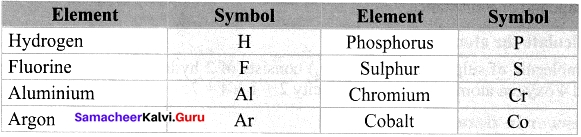Question 4.
Differentiate between elements and compounds.

 Elements Compounds An element is the simplest substance A compound is a chemical substance formed by the combination of two or more elements Elements combine to form compounds Compounds can be split into elements Atoms are the fundamental particle of an element Molecules are the fundamental particles of a compound

Question 5.
Write any five characteristics of compound.
Characteristics of compound:

1. A compound is formed only when the constituent elements combine in a fixed proportion.
2. The properties of a compound are different from those of its constituent elements.
3. A compound cannot be broken down by physical methods.
4. Compound is made up of different elements that are chemically combined.
5. A compound can be separated into its constituent elements by chemical methods only.

Question 6.
List comparative properties of metals and non-metals. Give three examples of each.

 Metals Non-Metals Metals are lustrous. They have a shiny Non metals are non lustrous. They surface have non- shiny surface Metals are generally hard Non-metals are generally soft Most metals are bendable Non-metals are non bendable Most metals can be bent, beaten into sheets and they can drawn into wires Non-metals are non ductile

Question 7.
Write down the properties of metalloids.
Properties of metalloids :

1. Metalloids usually look like metals but behave largely like non-metals.
2. Physically they are shiny, brittle solids with intermediate to relatively good electrical conductivity.
3. They can from alloys with metals.
4. Most of their physical and chemical properties are intermediate in nature, (v) They are semiconductors.
5. All are solids at room temperature.
6. Example : Germanium, Boron, Antimony, Silicon.

VII. Rewrite the sentence in correct form :

Question 1.
Elements contains two or more kinds of atoms and compounds contains only one kinds of atoms.
Elements contains two or more kinds of atoms and compounds contains two or more kinds of elements.

VIII. Higher Order Thinking questions :

Question 1.
Lists the metals, non-metals and metalloids which you used in your house, schools. Compare their properties.
Metals and their uses in our houses & schools :

1. Magnesium is used in the laboratory as magnesium ribbon.
2. Copper is used cooking utensils.
3. Zinc is mainly used as a protective coat for iron is our school and house gates.
4. Aluminum is used as cooking utensils and electrical cables which are used in our schools and houses.
5. Iron and steel are widely used is construction of house and school.
6. Sodium is used as a table salt and for flavoring, preserving food.
7. Coins are made up of nickel.
8. Lead is used in car batteries. Lead based alloys find extensive use in printing

Non-metals and their uses in houses & school:

1. Oxygen is essential for the breathing. It is used as oxidising agent in laboratory. Chlorine is used for purifying water.
2. Graphite is used in pencil leads.
3. Carbon is used as a fuel.
4. Bromine is used in dyes.
5. Iodine is used in laboratory to test for starch.

Metalloids and their uses in houses & schools :

1. Silicon is used in glass items.
2. Antimony is used in optical discs.
3. Tellurium is used in solar cells.
4. Boron is used in washing powders.
5. Germanium is used to make transistors which is used in laboratory in electrical devices.

Comparsion of properties of metals, non-metals & metalloids

 Metals Non-metals Metalloids They have lustre They are not lustrous Intermediate Conduct heat and electricity Poor conductors of electricity except graphite Intermediate (semi­conductors) They are malleable and ductile They are neither malleable nor ductile. Intermediate

Question 2.
Aakash noticed that the metal latch on gate was difficult to open during hot sunny days. However, this same latch was not difficult to open at night. Aakash observed that the latch and the gate are exposed to the sun during the day.
a) Formulate a hypothesis based on the information provided.
b) Briefly state how you would test the hypothesis stated in (a).
(a) Solids (Iron) expand on heating and contract on cooling. This causes the volume to matter of increase during heating or expansion, the mass of matter does not change.
(b) During heating the distance between the particles of the iron latch change. In an iron latch the distance between the iron particles increases when they gain enough heat. So during hot days the latch on the gate was difficult to open. At night, because of cooling the distance between the particles decreases. So at night it was not difficult to open the latch on the gate.

Question 3.
What changes take place in the movement and arrangement of particles during heating process?
When a substance is heated, its internal energy increases.

1. The movement of its particles increases.
2. Bonds between particles break when a substance melts evaporates. This causes the volume of matter to increase.
3. The closeness, arrangement and motion of the particles in a substance change when it changes state. But the size of the particles remain in same size, (i.e.) the mass of matter does not change.

Question 4.
In the diagram given below, the circle, square and triangle represent the atoms of different elements different elements.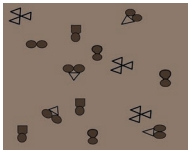Identify all combinations that represent.
a) A molecule of a compound
b) A molecule of an element consisting of two atoms
c) A molecule of an element consisting of three atoms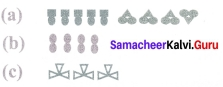IX. Assertion-reason questions :

Question 1.
Directions: Please refer to the following instructions:
A. Both statements are true and the 2nd statement is a correct explanation of the 1st statement.
b. Both statements are true but the 2nd statement is NOT a correct explanation of the 1st statement.
C. The 1st statement is false while the 2nd statement is true.
D. Both statements are false.

 1st Statement 2nd Statement 1. Oxygen is a element Oxygen cannot be broken down into anything simpler 2. Hydrogen is an element Hydrogen cannot be broken down into anything simpler 3. Air is a compound Air consists of carbon dioxide 4. Air is a mixture of elements only Only nitrogen, oxygen and neon gases exist in air 5. Mercury is solid in room temperature Mercury is a non-metal

1. (A) Both statements are true and the 2nd statement is a correct explanation of the 1st statement.
2. (A) Both statements are true and the 2nd statement is a correct explanation of the 1 st statement.
3. (D) Both statements are false.
Correct statement:

1. Air is a mixture of gases.
2. Air consists of Nitrogen Oxygen, CO,, other noble gases.

4. (A) Both statements are true and the 2nd statement is a correct explanation of the 1 st statement.
5. (D) Both statements are false.
Correct statement:

1. Mercury is liquid at room temperature.
2. Mercury is a metal.

### Samacheer Kalvi 7th Science Matter Around Us Intext Activities

Activity – 1
Complete the following table by writing compounds of its constituents.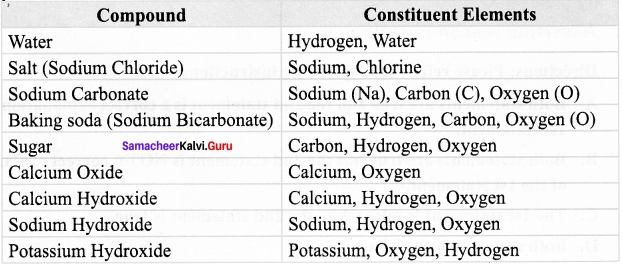Activity – 2
Complete the following table by counting the number of different elements in a compounds and give appropriate name.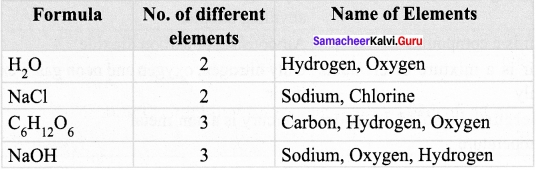Activity – 3
Write down the symbols of the following elements.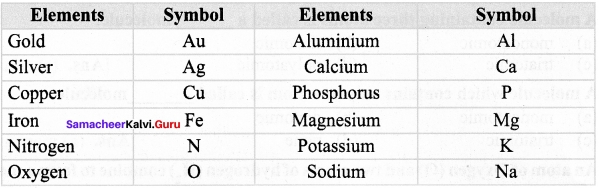Activity – 4
Write down atomicity of the following elements and compounds

### Samacheer Kalvi 7th Science Matter Around Us Additional Questions

Question 1.
The graphite refill used is pencil is made of element called __________
(a) graphite
(b) carbon
(c) silicon
(d) sodium
(b) carbon

Question 2.
The smallest unit of an element is __________
(a) atom
(b) molecule
(c) compound
(d) none
(a) atom

Question 3.
The most abundant type of atom is the universe is __________
(a) Helium
(b) Hydrogen
(c) Argon
(d) Argon
(b) Hydrogen

Question 4.
__________ can be formed by the same or different kinds of atoms.
(a) Atom
(b) Molecule
(c) Gases
(d) None
(b) Molecule

Question 5.
__________ is a substance that is made up of three oxygen atoms chemically combined.
(a) Oxygen
(b) Hydrogen
(b) Ozone
(d) CO2
(a) Oxygen

Question 6.
A molecule containing three atoms is called a __________ molecule
(a) monoatomic
(b) diatomic
(c) triatomic
(d) polyatomic
(c) triatomic

Question 7.
A molecule which contains only one atom is called __________ molecule
(a) monoatomic
(b) diatomic
(c) triatomic
(d) none
(a) monoatomic

Question 8.
An atom of Oxygen (O) and two atoms of hydrogen (H.) combine to form a molecule of __________
(a) hydrogen
(b) ozone
(c) water
(d) none
(c) water

Question 9.
__________ is the first scientist who used the term element.
(a) New ton
(b) Einstein
(c) Robertr boyle
(d) Robert hook
(c) Robertr boyle

Question 10.
Matter is its simplest form is called __________
(a) molecule
(b) Metals
(c) element
(d) none
(c) element

II. Fill in the blanks :

1. The name copper was taken from ______
2. The first letter of a symbol of an element is always written as a the second letter as a ______ letter and the second letter as a _____ letter
3. There are ______ hydrogen atoms in water molecule.
4. There is ______ oxygen atom is water molecule.
5. ______ is the total number atoms present in one molecule of an element, compound or a substance.
6. ______ is a mixture of gases
7. ______ is the life-giving element is the air
8. During heating or expansion, the ______
9. ______ is the smallest particle of an element.
10. ______ of an element consist of a fixed number of different types of atom.

1. cypress
2. capital,small
3. 2
4. 1
5. atomicity
6. Air
7. Oxygen
8. mass
9. Atom
10. Molecules

III. Write true or false :

Question 1.
Metals are usually solids or gases at room temperature.
False. Metals are usually solids at room temperature.

Question 2.
Non-metals are non-malleable.
True.

Question 3.
Non-metals are usually good conductors of heat and electricity.
(False) Metals are usually good conductors of heat and electricity.

Question 4.
Sodium chloride is used as table salt and for flavoring and preserving food.
True.

Question 5.
Lead is also known as plumbum.
True

IV. Match the following :

Question 1.

 1 Co2 (a) Robert Boyle 2 Sulphur (b) Making mobile phones 3 Element (c) triatomic molecule 4 Gallium (d) Polyatomic molecule

1. c
2. d
3. a
4. b

Question 2.

 1 Non-metals (a) Silicon 2 Metals (b) fire extinguisher 3 Metalloids (c) dull and soft 4 Oxygen (d) hard and shiny 5 Water (e) supports fire

1. c
2. d
3. a
4. b

V. Give very short answer :

Question 1.
How many elements are known at present?
118.

Question 2.
Name the element which is used for making computer chips.
Silicon

Question 3.
Name the constituents of water.
Hydrogen and Oxygen.

Question 4.
What is the expansion of IUPAC.
International Union of Pure and Applied Chemistry.

Question 5.
What is the Latin name of Sodium?
Natrium

Question 6.
What is a molecule?
Molecule is the smallest unit of compound.

Question 7.
What is the fundamental particle of an element?
Atom.

Question 8.
What is a chemical formula?
A chemical formula is a symbolic representation of one molecule of an element or a compound.

Question 1.
What is an atom?
The smallest unit of an element that exhibits the properties of the element is called as ‘atom’.

Question 2.
What are the 3 most abundant atoms on the earth?
On earth the three most abundant atom are iron, oxygen, and silicon.

Question 3.
Write any two classification of molecule.

1. A molecule which contains only one atom is called monatomic molecule (inert gases)
2. A molecule which contains two atoms is called diatomic molecule (oxygen, nitric oxide, hydrogen, etc.).

Question 4.
Classify the following as element and compound, (i) Silver (ii) Ammonia (iii) Water (iv) Mercury.

1. Silver – element
2. Ammonia – compound
3. Water – compound
4. Mercury – clement

Question 5.
Give an account of elements known to us.
There are 118 known elements till date. 94 of these elements occur naturally while 24 elements have been created artificially in the laboratory.

Question 6.
What are metalloids? Give examples.
Metalloids are the elements which have properties intermediate, between those of metals and non-metals. Ex.: Boron, Silicon, Germanium, Arsenic, Antimony.

Question 7.
Give an example of a metal which
(a) is liquid at room temperature.
(b) is the best conductor of heat.
a) Mercury
b) Silver, Copper

Question 8.
Write any two properties of a compound.

1. The properties of a compound are different from those of its constituent elements.
2. A compound cannot be broken down by physical methods. This is because a compound is made up of different elements that are chemically combined. Sodium chloride cannot be separated by physical methods such as filtration.

Question 9.
What happens to matter during heating?

1. SOLID : When solid is heated, the particles gain energy and vibrate more vigorously.
2. LIQUID : Melting occurs when the melting point is reached. The solid changes to its liquid state.
3. LIQUID : When a liquid is heated the particles gain energy and vibrate more
4. GAS : Boiling occurs when the boiling point is reached. The liquid changes to its gaseous State.

Question 10.
How do hot air balloons float?
When air inside the hot air balloon is heated with a burner, it expands. The expansion causes the density of the air inside the balloon to decrease. Hence, the air inside the balloon has a lower density that the air outside of the balloon. This difference is density allows the hot-air balloon to float.

VII. Complete the table

Question 1.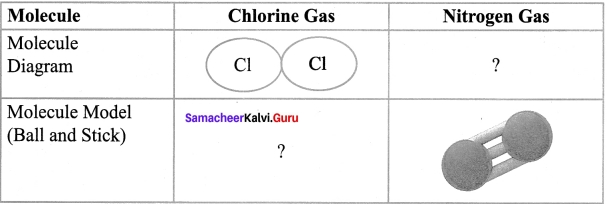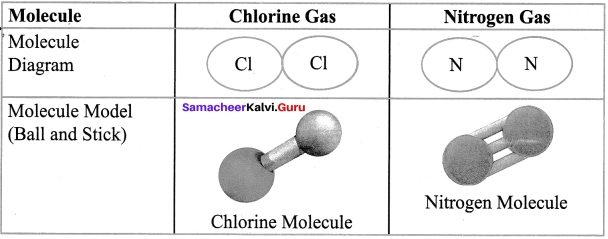Question 2.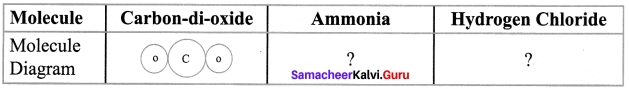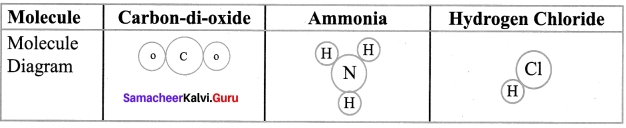Question 3.

 Compound Constituent elements Sodium carbonate Sodium, Carbon. Oxygen Water (i) ? Calcium oxide (ii) ? Sugar Carbon, Hydrogen, Oxygen

1. Hydrogen, Oxygen
2. Calcium, Oxygen.

Question 4.
Complete the table by writing the symbols of the following elements

 Element Symbol Aluminium Al Nickel Bromine Helium Magnesium

 Element Symbol Aluminium Al Nickel Ni Bromine Br Helium He Magnesium Mg

Question 5.
Complete the table:

 Element Latin Name Symbol Mercury – Hg Lead Plumbum – Iron – Fe Potassium Kalium –

1. Hydrargyrum
2. Pb
3. Ferrum
4. K

Question 1.

1. Draw the symbol for some elements as proposed by Dalton.
2. What are rules to be followed while assigning symbol to element.

1. Symbol for some elements as proposed by Dalton.
2. The following rules are followed while assigning symbol to an elements: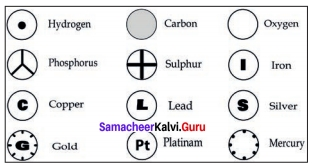(a) Chemical symbols usually consist of one or two letters
(b) The symbols of most elements correspond to the first letter (which is capitalized) of their English name. For example, the symbol for oxygen is “O” and that for hydrogen is “H”.

Higher Order Thinking questions : (HOTS)

Question 1.
Why is hydrogen considered as an element?
Hydrogen is considered as an element because,

1. Hydrogen cannot be broken into new substance by ordinary physical or chemical means, (i.e) by applying heat, light.
2. Hydrogen has one kind of atoms.

Question 2.
Why is water considered as compound?